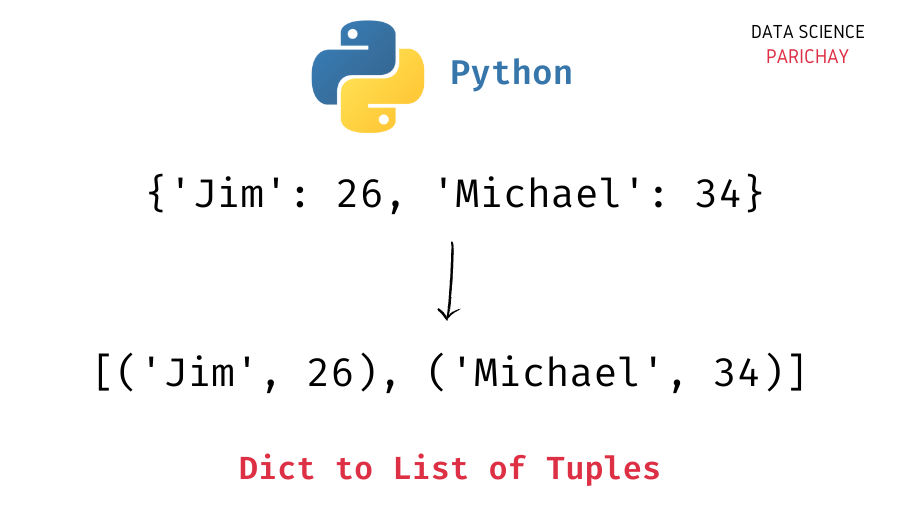# Convert Dictionary to List of Tuples in Python

In this tutorial, we will look at how to convert a Python dictionary to a list of (key, value) tuples. For example, you have a dictionary `{key1: value1, key2: value2}` and you want to get the (key: value) tuple pairs in a list – `[(key1, value1), (key2, value2)]`.

There are multiple ways to get a list of (key, value) tuple pairs from a dictionary. Some of which are –

1. You can use the dictionary’s `.items()` function and the `list()` function.
2. Use a loop to iterate over the dictionary items and add the (key, value) tuple pairs to a list.
3. Using list comprehension to construct the list of (key, value) tuple pairs.

Let’s look at examples of using the above methods to get a list of tuples from a dictionary.

You can use a combination of the dictionary `items()` function and the `list()` function to convert a dictionary to a list of (key, value) tuples.

```# create a dictionary
age_dict = {'Sam': 26, 'Emma': 21, 'Varun': 29, 'Zayn': 24}
# dictionary to list of tuples
age_ls = list(age_dict.items())
print(age_ls)```

Output:

`[('Sam', 26), ('Emma', 21), ('Varun', 29), ('Zayn', 24)]`

We get the desired list of (key, value) tuple pairs. The dictionary `items()` function returns a view object containing the (key, value) tuple pairs, and the `list()` function converts the view object to a list.

Here we use a loop to iterate over the items in the dictionary and then append the (key, value) tuple to our result list.

```# create a dictionary
age_dict = {'Sam': 26, 'Emma': 21, 'Varun': 29, 'Zayn': 24}
# dictionary to list of tuples
age_ls = []
for key,val in age_dict.items():
age_ls.append((key, val))
print(age_ls)```

Output:

📚 Data Science Programs By Skill Level

Introductory

Intermediate ⭐⭐⭐

🔎 Find Data Science Programs 👨‍💻 111,889 already enrolled

Disclaimer: Data Science Parichay is reader supported. When you purchase a course through a link on this site, we may earn a small commission at no additional cost to you. Earned commissions help support this website and its team of writers.

`[('Sam', 26), ('Emma', 21), ('Varun', 29), ('Zayn', 24)]`

We get the same result as above.

The above method can be reduced to a single line using list comprehension.

```# create a dictionary
age_dict = {'Sam': 26, 'Emma': 21, 'Varun': 29, 'Zayn': 24}
# dictionary to list of tuples
age_ls = [(key, val) for key,val in age_dict.items()]
print(age_ls)```

Output:

`[('Sam', 26), ('Emma', 21), ('Varun', 29), ('Zayn', 24)]`

We get the same results as above. Here, we use list comprehension to build a list of (key, value) tuple pairs from the dictionary’s items.

You might also be interested in –

•